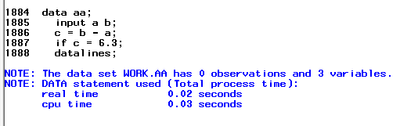## SAS giving wrong results

Hi,

data aa;

input a b;
c = b - a;
if c = 6.3;
datalines;
2.9 9.2
;
run;Mathematically c should have been 6.3 (9.2 - 2.9), however, the above code is giving zero observation as the results is not equal to 6.3 at some decimal level.

I have tried the same code for the following differences and similar issues were observed.

2.01 - 1.02

5.4 - 4.5 ...

Have anyone faced similar issues?

2 REPLIES 2

## Re: SAS giving wrong results

Absolutely! This has been discussed in several posts and here's a great solution to read through.

If you want to see what's going on behind the scenes, display your calculated value (b-a) and the value you wish to compare against (let's call this d) in their hexidecimal representation using the HEX format, like so:

``````data aa;
input a b;
c = b - a;
d = 6.3;

put c hex16.;
put d hex16.;

datalines;
2.9 9.2
;
``````

You can see that the two values are very similar, except for the end due to rounding errors. SAS Documentation covers this topic here: https://documentation.sas.com/doc/en/pgmsascdc/v_037/lepg/n0o6e1t72yad42n10y9wf8pgmat8.htm#p0lghy68t...

For your example, the ROUND function will do the trick like so:

``````data aa;
input a b;
c = b - a;
d = 6.3;

if round(c,.1) = d;

datalines;
2.9 9.2
;
``````

## Re: SAS giving wrong results

Hi @amalap and welcome to the SAS Support Communities (belatedly)!

Sorry to see that your question had not been answered for a couple of days. This is very unusual for this rather elementary type of question, so I suspect that some technical delay must have prevented the forum experts and myself from seeing your post early.

Now you have already received a link to a relevant page of the documentation and the practical solution of using the ROUND function.

Let me just add a few bits (pun intended) to explain the "wrong results" which you observed in more detail.

The numbers in your first example, 9.2 and 2.9, have one decimal place each. Any such integer multiple of one tenth with a decimal place other than 0 or 5 is affected by numeric representation error, which means that the number's internal binary floating-point representation used by SAS (and not only SAS) to store numeric values is not 100% exact due to rounding. Indeed, the decimal equivalents of the internal 64-bit representations of 9.2 and 2.9 under Windows or Unix are

```9.199999999999999289457264239899814128875732421875
```

and

`2.899999999999999911182158029987476766109466552734375`

respectively. Well, you need to be lucky to get perfect results from a calculation starting with inexact values like these. As we will see below, this example is actually close to such a "lucky" case.

Written in the binary system, both 9.2 and 2.9 are periodic fractions:

```1001.0011001100110... (mathematical binary representation of 9.2)
10.1110011001100... (mathematical binary representation of 2.9)```

where the 4-digit patterns 0011 and 1100, respectively, are repeating infinitely often. Given the limited storage space for a numeric variable (of length 8 bytes), these numbers are rounded in order to obtain the best approximation that fits into 53 bits: the so called "implied bit" for the trivial leading 1 and the 52 mantissa bits (see documentation) for the remaining binary digits (of integer and fractional part). The remaining 64−52=12 bits of storage space accommodate the sign and the exponent (i.e. the order of magnitude).

```put(9.2, binary64.)='0100000000100010011001100110011001100110011001100110011001100110'
put(2.9, binary64.)='0100000000000111001100110011001100110011001100110011001100110011'```

Note that both numbers were rounded off because the next digit was zero, hence the slightly "too small" decimal equivalents.

For the subtraction (which can be done manually like in elementary school, the repeating patterns reducing the effort considerably), the representation of 2.9 is adapted to the exponent of the larger number: basically, both numbers are now written as multiples of 2**3=8.

``` 1.0010011001100110011001100110011001100110011001100110
-0.010111001100110011001100110011001100110011001100110011
=0.110010011001100110011001100110011001100110011001100101```

Thanks to the 80 bits available to the processor, the right shift of 2.9 by two bits does not lead to additional truncation or rounding. As a consequence, however, the rightmost bit 1 hits a zero bit from 9.2 which was due to rounding for the binary64. representation in the previous step, and this causes the damage. (There is still a bit of hope at this point, though, because of the rounding in the final step, described below.)

Finally, the result is "normalized," i.e., written as 1.10010011001... * 2**2 (again, a periodic fraction, the repeating pattern being 1001) and rounded to 52 mantissa bits. Only the very last "1" does not fit into the mantissa, so this is a borderline case like ".5" in the decimal system! Rounding up would lead to the correct result (the internal representation of 6.3), but -- unfortunately in this case -- the rule in these borderline situations is "round to the even number," i.e., ending in 0. So the result is the internal representation

`0100000000011001001100110011001100110011001100110011001100110010`

with that rounding error in the last bit making it slightly less than the internal representation of 6.3. (Which, of course, ends in ...10011, correctly rounding the infinite sequence of 1001's.)

Your other two examples can be explained in exactly the same way. The case of 5.4 − 4.5 is particularly easy because, unlike all the other numbers, 4.5 (ending in .5) has an exact, finite binary representation -- 100.1 --, so you'll subtract many trailing zeros in the manual calculation.

Discussion stats
• 2 replies
• 173 views
• 2 likes
• 3 in conversation